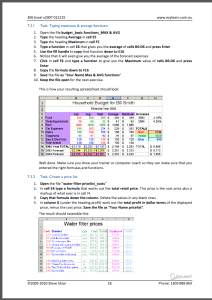Sale!

Microsoft Excel Intermediate Course 306 – Functions & Cell Referencing

\$69.00 \$49.00 inc GST

• Number & percentage formatting: Use percentage formatting to better use them in formulas and functions
• Understand cells formatting (even without data in them)
• Relative & Absolute Cell references: How relative cell referencing works when copying formulas & functions quickly (and when they don’t work and why)
• Use Absolute cell referencing to reduce the amount of calculations in a spreadsheet
• Learn and understand fundamental mathematical functions (skills that make more complicated functions easier to understand)
• Combine two formulas into one and write better formulas and get to know the order of mathematical calculations
• Using Functions like Maximum, Minimum and average
• Understand the “Insert Functions dialog box”,  search for functions and understand “arguments” (or syntax) in functions and function categories such as financial, Date and time, maths and trig
• Understanding and constructing an IF Statement
• Comments: Uses for comments. Insert comments to explain the purpose of a cell, choose size and location of the comments and control the way they appear on the spreadsheet; edit comments & change comment formatting
• Show/Hide Columns/Rows: hide columns or rows with confidential information. Print the spreadsheet without the confidential information.

Description

• Number and percentage formatting: Understanding the various ways of formatting, including the understanding form percentages to better use them in formulas and functions.
• Understand that cells can be formatted (even without data in them) so that when you enter data, it can look completely different to what you expected to see. Sometimes cells can be formatted in such a way that even though you enter numbers, a date may be shown. Learn about why in this section.
• Relative and Absolute Cell references: Understand how relative cell referencing works to help you copy formulas and functions quickly. Also understand where they don’t work and why. Absolute cell referencing is a powerful way to reduce the amount of calculations you have to create and can save several columns or rows of typing. Learn the different types of and how to use absolute cell references in this section.
• Learn and understand some fundamental mathematical functions that will help you perform most formulas and functions. These are fundamental skills that will help make more complicated functions easier to understand.
• Understand how to combine two formulas into one and as a result write much better formulas and get to know the order of mathematical calculations.
• Using Functions like Maximum, Minimum and average
• Getting to know the “Insert Functions dialog box”, how to search for a particular function and understand the concept of “arguments” (or syntax) in functions and function categories such as financial, Date and time, maths and trig, etc.
• Understanding and constructing an IF Statement.
• Comments: Understand the uses for comments. Insert comments that help to explain the purpose of a cell, choose the size and location of the comments and control the way they appear on the spreadsheet. Edit comments and change the formatting within a comment, change the name of the person who made the comment, and make sure that the comment appears all the time or only when you move the mouse to it.
• Show/Hide Columns/Rows: Learn how to hide columns or rows that contain confidential information. This way you can print the spreadsheet out without the confidential information, but use the confidential information to perform the necessary calculations.

Reviews

There are no reviews yet.

Only logged in customers who have purchased this product may leave a review.